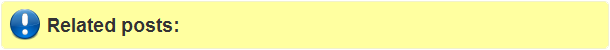## Monday, June 10, 2013

### How to get raster pixel values along the overlaying line?

One afternoon at Java City, my friend Eric and I were discussing about the ways to to get raster pixel values along the overlaying line. The conversation encourages me to write an quick and dirty solution to solve the issue. The following R code snippet helps to conceive an idea to extract the raster values which are intersect or touch by the overlaying straight line in easy fashion using R raster package.

#Print the raster pixel values along the overlaying line in R. The line's start and end row/col (coordinates) must be provided.

library(raster)
#Create an arbitrary raster, for instance I used a names of color as raster pixel values.
r <- as.raster(matrix(colors()[1:100], ncol = 10))

#Start coordinate of a sample line
x0=1      #row = 1
y0=3      #column = 3

r[x0,y0]

#End coordinate of a sample line
x1=10        #row =10
y1=7 #column=7

#Easy sample line generation algorithm : A naïve line-drawing algorithm

dx=x1-x0
dy=y1-y0

for( x in x0:x1)
{
y = y0 + (dy) * (x - x0)/(dx)

#Print the raster pixel values along the line
print(r[x,y])

}

Pretty simple concept. You can tweak the code & the line drawing algorithm as your requirement. There are several line drawing algorithm available in the internet. Here I used a simplest one that I found.

Input:

Output:
The raster pixel values that intersect by a line between(x0,y0) &(x1,y1).

Pixel values: